# Algebra II : Percentiles

## Example Questions

### Example Question #1 : Percentiles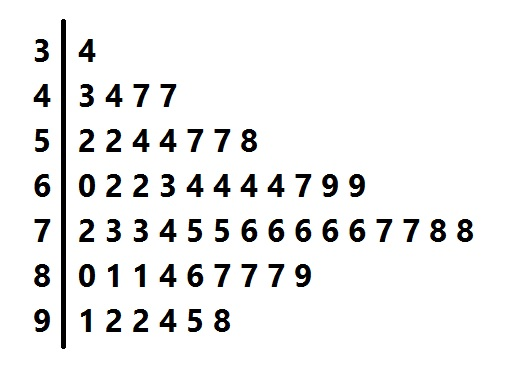Above is the stem-and-leaf display for a group of test scores. At what percentile would the student who made a score of 86 be?

Possible Answers: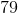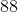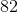None of the other responses is correct.

Correct answer:Explanation:

The stem-and-leaf display represents 53 scores. The "stems" (or left column) represent the tens digits, and the "leaves" in each row represent units digits, so the scores are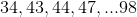The student who scored an 86 outscored 42 of the students, or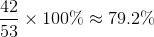of them. Since percentile is given to the nearest whole number, the correct response is 79.

### Example Question #2 : Percentiles

Find the 85th percentile score in the following test results.

{95, 88, 70, 75, 83, 70, 66, 91, 68, 76, 82}

Possible Answers: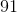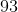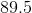Correct answer:Explanation:

{95, 88, 70, 75, 83, 70, 66, 91, 68, 76, 82}

Arrange the values in numerical order.

{66, 68, 70, 70, 75, 76, 82, 83, 88, 91, 95}

Use the following to calculate percentile-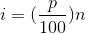whereis the percentile andis the number of data in the set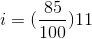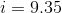This number gives of the location of the 85th percentile in our ordered set. Since it is not a whole number, round up, which will give us 10.

The 85th percentile score is the 10th value in our set, which is 91.

### Example Question #3 : Percentiles

If a student is ranked eight out of ten in a competition, what is the student's percentile rank?

Possible Answers: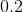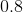Correct answer:Explanation:

The formula to calculate a percentile rank is: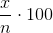whererepresents total of scores below the rank, andis the total number of competitors.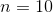Substitute the values into the formula.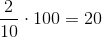### Example Question #4 : Percentiles

The times for a six-mile race are shown below.

Sean: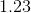hours

Ankur: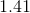hours

Sarah: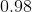hours

Fred: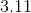hours

Ron: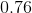hours

Jareth: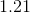hours

Determine which percentile Sean's time is in.

Possible Answers: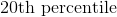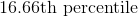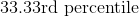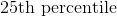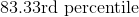Correct answer:Explanation:

First arrange the runners in order of fastest to slowest times:

Ron

Sarah

Jareth

Sean

Ankur

Fred

There are six runners so there will be six different percentiles, each seperated by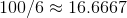percent. A person's percentile refers to which percentage of times are below that person's time. For example, Ron's time is better than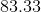percent of all runners, so he is in therd percentile.

Then each runner is in the following percentiles:

Ron -rd

Sarah -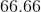th

Jareth -th

Sean -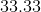rd

Ankur -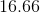th

Fred -th

As you can see, Sean is in therd percentile.

### Example Question #5 : Percentiles

In a litter of nine kittens, the only orange kitten weighs more than three in the litter, and less than the other remaining five.

What is the percentile of the orange kitten's weight in comparison to the litter?

Possible Answers: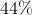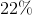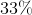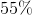Correct answer:Explanation:

The percentile is equal to how many items in the set are equal to or less than the one in question divided by the total number of items in the set.

Because there are four items equal to or less than the orange kitten's weight and there are nine items total in the set, the percentile is equal to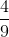or### Example Question #6 : Percentiles

98, 99, 99, 100, 101, 102, 104, 104, 105, 105, 107, 110, 112, 112

For the above data set, 102 is in what percentile?

Possible Answers:

57th

43rd

1st

50th

Correct answer:

43rd

Explanation:

In the data set, 102 is the 6th data point listed. There are 14 data points total. This means that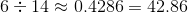% of the data is at or below 102. That's approximately the 43rd percentile.

### Example Question #7 : Percentiles

98, 99, 99, 100, 101, 102, 104, 104, 105, 105, 107, 110, 112, 112

For the above data set, 110 is in what percentile?

Possible Answers:

90th

75th

14th

86th

Correct answer:

86th

Explanation:

The number 110 is the 12th in the list of 14 data points. That means that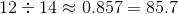%. Since about 86% of the data points are at or below 110, it's in the 86th percentile.

### Example Question #8 : PercentilesAbove is the stem-and-leaf display for a group of test scores. Which of the following scores would come closest to being at the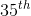percentile?

Possible Answers: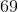Correct answer:Explanation:

The stem-and-leaf display represents 53 scores. The score at the 35th percentile would be the score that is greater than 35% of the scores, or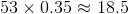scores.

Rounding, we count 19 scores from the bottom: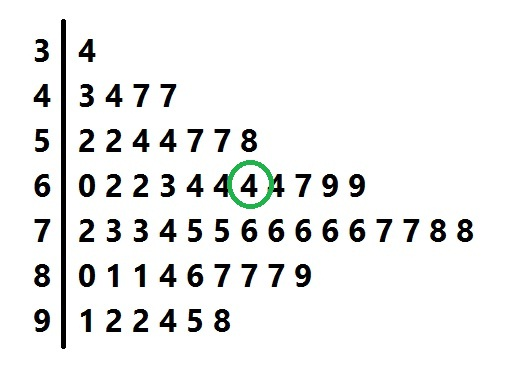The score represented is 64, which is the correct response.

### All Algebra II Resources